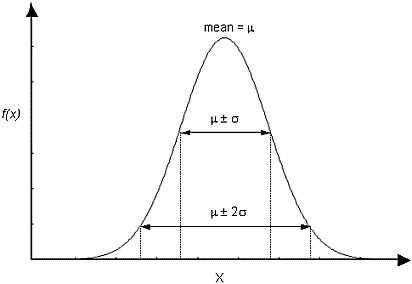The Rocscience International Conference 2021 Proceedings are now available. Read Now

# Normal Distribution

The Normal (or Gaussian) distribution is the most common type of probability density function (PDF), and is generally used for probabilistic studies in geotechnical engineering. Unless there is a good reason to use one of the other PDFs available in RocSupport, it is recommended that the user choose the Normal distribution.

For a Normal distribution, about 68% of observations should fall within one standard deviation of the mean, and about 95% of observations should fall within two standard deviations of the mean. Nearly all observations (> 99%) should fall within 3 standard deviations of the mean.

Normal probability density function, showing standard deviation ranges## Truncated Normal Distribution

A truncated Normal distribution can be defined by setting the desired minimum and/or maximum values for the variable. For practical purposes, if the minimum and maximum values are at least 3 standard deviations away from the mean, you will obtain a complete normal distribution. If the minimum / maximum values are less than 3 standard deviations away from the mean, the distribution will be visibly truncated.